# Ellipse

(diff) ← Older revision | Latest revision (diff) | Newer revision → (diff)

(real)

A planar curve obtained by the intersection of a circular cone with a plane not passing through the vertex of the cone and intersecting all its generators in points of one of its sheets. An ellipse also turns out to be the set of pointsof a plane (see Fig.) for each of which the sum of the distances from two given points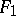and(the foci) is constant and equal to. The distance between the foci is called the focal distance and is usually denoted by. The mid-point of the segmentis called the centre of the ellipse.Figure: e035390a

The line on which the foci of the ellipse lie is called the first (or focal) axis. The line through the centre of the ellipse and perpendicular to the first axis is called the second axis. The axes of the ellipse are its axes of symmetry. The points of intersection of the ellipse with the axes of symmetry are called its vertices. The major axis of the ellipse is the segment (and also its length) of the first axis between the vertices. The minor axis is the segment (and also its length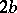) of the second axis between the vertices. The number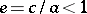is called the eccentricity of the ellipse. A diameter of the ellipse is any straight line passing through the centre; a diameter can also be defined as a straight line passing through the mid-points of parallel chords. The directrix of the ellipse corresponding to a given focus(the associated directrix) is the lineperpendicular to the first axis at distance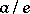from the centre. An ellipse has in general two directrices. An ellipse is a central second-order curve with canonical equation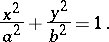The equation of the tangent to an ellipse at a pointis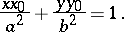The focal parameterof an ellipse (half the length of the chord passing through a focus and perpendicular to the first axis) is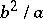. By means of the focal parameter one can write the equation of an ellipse in the formwhereandare polar coordinates,.

If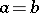, the ellipse becomes a circle,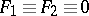is its centre,its radius,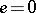, and there is no directrix.

An ellipse has the following optical property: A light ray emanating from one focus passes through the other focus after mirror reflection in the ellipse.

A second-order curve with canonical equation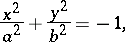whereandare real numbers, is called an imaginary ellipse.